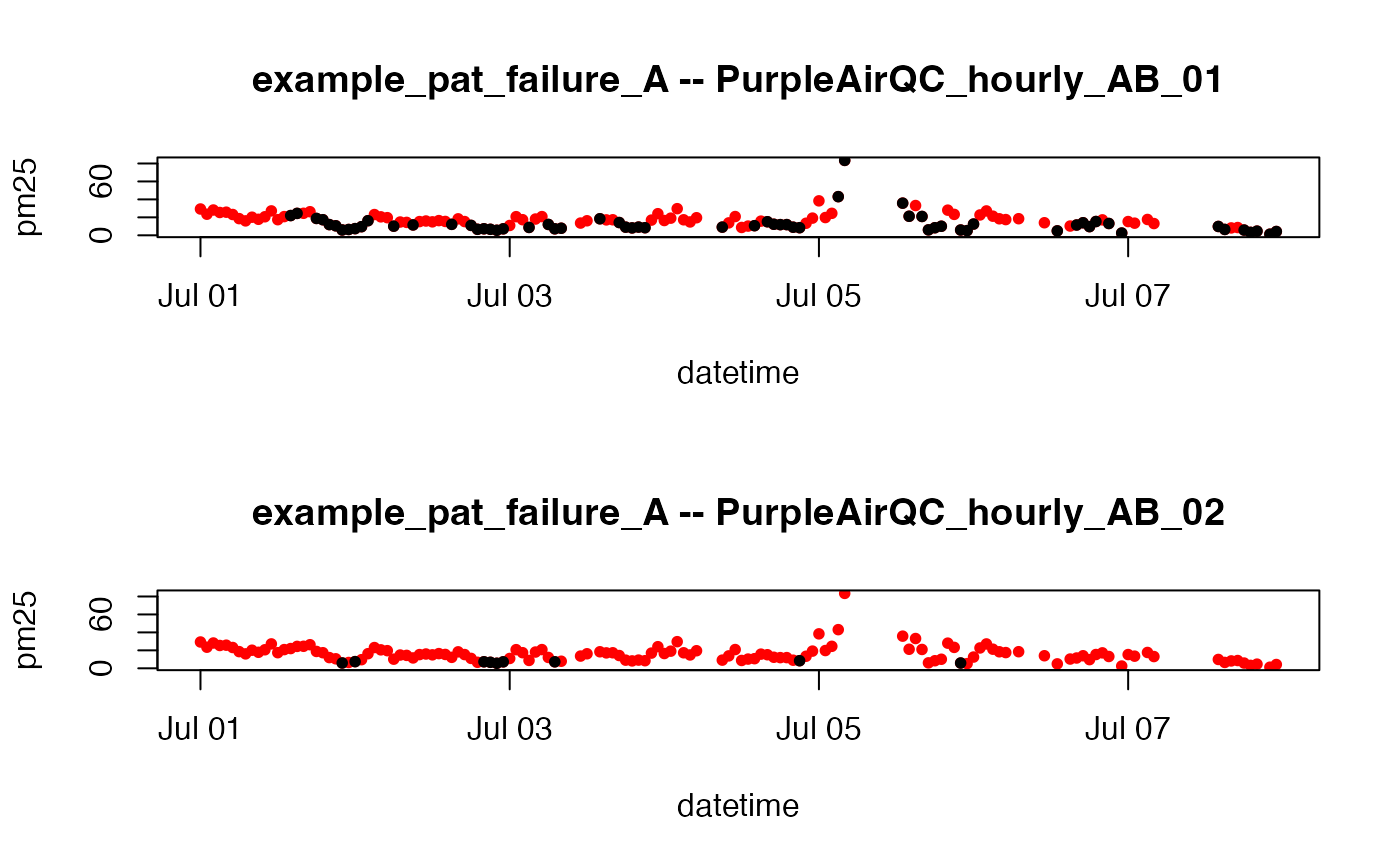Creates a pm25 timeseries by averaging aggregated data from the A and B channels and applying the following QC logic:

1. Create pm25 by averaging the A and B channel aggregation means

2. Invalidate data where: (min_count < 20)

3. Invalidate data where: (p-value < 1e-4) & (mean_diff > 10)

4. Invalidate data where: (pm25 < 100) & (mean_diff > 20)

PurpleAirQC_hourly_AB_01(pat = NULL, min_count = 20, returnAllColumns = FALSE)

## Arguments

pat A PurpleAir timeseries object. Aggregation bins with fewer than min_count measurements will be marked as NA. Logical specifying whether to return all columns of statistical data generated for QC algorithm or just the final pm25 result.

## Value

Data frame with columns datetime and pm25.

## Note

Purple Air II sensors reporting after the June, 2019 firmware upgrade report data every 2 minutes or 30 measurements per hour. The default setting of min_count = 20 is equivalent to a required data recovery rate of 67

## Examples

# \donttest{
library(AirSensor)

df_00 <-
example_pat_failure_A %>%
pat_qc() %>%
PurpleAirQC_hourly_AB_00()

df_01 <-
example_pat_failure_A %>%
pat_qc() %>%
PurpleAirQC_hourly_AB_01()

df_02 <-
example_pat_failure_A %>%
pat_qc() %>%
PurpleAirQC_hourly_AB_02()

layout(matrix(seq(2)))

plot(df_00, pch = 16, cex = 0.8, col = "red")
points(df_01, pch = 16, cex = 0.8, col = "black")
title("example_pat_failure_A -- PurpleAirQC_hourly_AB_01")

plot(df_00, pch = 16, cex = 0.8, col = "red")
points(df_02, pch = 16, cex = 0.8, col = "black")
title("example_pat_failure_A -- PurpleAirQC_hourly_AB_02")layout(1)
# }